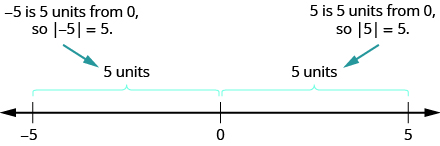## Simplifying Expressions With Absolute Value

### Learning Outcomes

• Use correct notation to indicate absolute value
• Simplify expressions that contain absolute value
• Evaluate expressions that contain absolute value

We saw that numbers such as $5$ and $-5$ are opposites because they are the same distance from $0$ on the number line. They are both five units from $0$. The distance between $0$ and any number on the number line is called the absolute value of that number.

Because distance is never negative, the absolute value of any number is never negative.

The symbol for absolute value is two vertical lines on either side of a number. So the absolute value of $5$ is written as $|5|$, and the absolute value of $-5$ is written as $|-5|$ as shown below.### Absolute Value

The absolute value of a number is its distance from $0$ on the number line.
The absolute value of a number $n$ is written as $|n|$.

$|n|\ge 0$ for all numbers

### example

Simplify:

1.  $|3|$
2.  $|-44|$
3.  $|0|$

Solution:

 1. $|3|$ $3$ is $3$ units from zero. $3$
 2. $|-44|$ $−44$ is $44$ units from zero. $44$
 3. $|0|$ $0$ is already at zero. $0$

### try it

In the video below we show another example of how to find the absolute value of an integer.

We treat absolute value bars just like we treat parentheses in the order of operations. We simplify the expression inside first.

### example

Evaluate:

1. $|x|\text{ when }x=-35$
2. $|\mathit{\text{-y}}|\text{ when }y=-20$
3. $-|u|\text{ when }u=12$
4. $-|p|\text{ when }p=-14$

Notice that the result is negative only when there is a negative sign outside the absolute value symbol.

### example

Fill in $\text{< },\text{ > },\text {or }=$ for each of the following:

1. $|-5|$   ___  $-|-5|$
2. $8$  ___  $-|-8|$
3. $-9$  ___  $- |-9|$
4. $-|-7|$  ___  $- 7$

### try it

In the video below we show more examples of how to compare expressions that include absolute value and integers.

Absolute value bars act like grouping symbols. First simplify inside the absolute value bars as much as possible. Then take the absolute value of the resulting number, and continue with any operations outside the absolute value symbols.

### example

Simplify:

1. $|9 - 3|$
2. $4|-2|$

### example

Simplify: $|8+7|-|5+6|$.

### example

Simplify: $24-|19 - 3\left(6 - 2\right)|$

### try it

Watch the following video to see more examples of how to simplify expressions that contain absolute value.